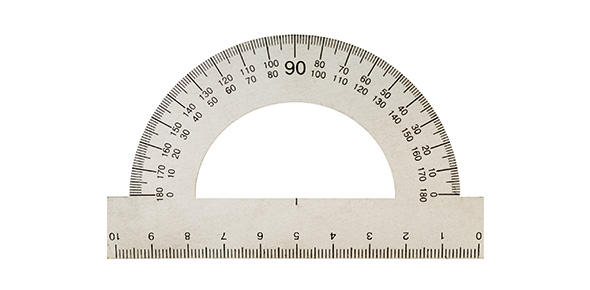# What Do You Know About Measuring Length?

8 Questions | Total Attempts: 104SettingsYear 9 measurement quiz. Includes perimeter and circumference.

• 1.
If I was going to measure the distance from Richmond to Newcastle, which unit of measurement should I choose?
• A.

Centimetres

• B.

Kilometres

• C.

Millimetres

• D.

Decametres

• E.

Metres

• 2.
Which would be the correct unit of measurement to choose for measuring the length on an ant?
• A.

Kilometres

• B.

Metres

• C.

Millimetres

• D.

Centimetres

• 3.
To convert centimetres to metres I do the following.
• A.

Divide by 100.

• B.

Multiply by 10.

• C.

Divide by 10.

• D.

Divide by 100.

• 4.
4000 mm equals ____________ cm
• 5.
Write a sentence to describe what perimeter means.
• 6.
What is the special name for the perimeter of a circle?
• 7.
Choose the correct method for calculating the circumference of a circle with a diameter of 10 cm
• A.

5 times Pi squared.

• B.

5×π

• C.

10×π

• D.

10 squared time Pi

• 8.
A farmer has a rectangular paddock with length of 25 m and breadth of 15 m. What is the perimter of this paddock?
Related TopicsBack to top